# Patient Experience survey of GP and local nhs services 2011/12 Volume 1: Technical Report

Scottish Patient Experience Survey of GP and Local NHS Services 2011/12. This is a postal survey which was sent to a random sample of patients who were registered with a GP in Scotland in October 2011. This report contains details the survey design and development.

### Annex E: Significance Testing

a. Comparison of results at National, NHS Board and CHP over the two survey periods (2009/10 and 2011/12)

For each question (where comparison is possible), the test statistic can be calculated as follows: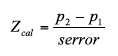For a result in 2011/12 to be not statistically significantly different from 2009/10, then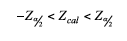To illustrate this, let the:

• percentage positive score in 2009/10 and 2011/12 for Scotland, an NHS Board or CHP be denoted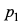by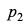and respectively.
• number of valid responses in 2009/10 and 2011/12 be denoted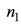by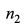and respectively.
• design effect in years 2009/10 and 2011/12 be denoted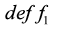as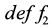and respectively.

Then the standard error can be calculated as: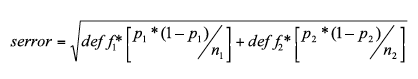b. Comparison of results for NHS Board and CHP with Scotland

For each question (where comparison is applicable), the test statistic can be calculated as follows: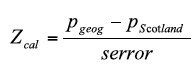For the NHS Board or CHP to be not statistically significantly different from Scotland results, then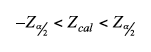To illustrate this, let the:

• percentage positive score for NHS Board or CHP be denoted by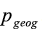.
• percentage positive score for Scotland be denoted by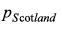.
• number of valid responses for be denoted by NHS Board or CHP (geography) by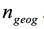.
• number of valid responses for Scotland be denoted by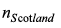.
• design effect for NHS Board or CHP and Scotland be denoted by and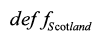respectively.

Then the standard error can be calculated as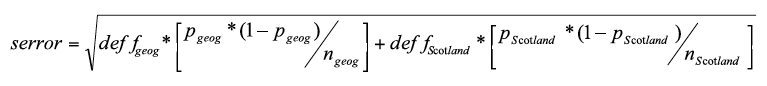### Contact

Email: Gregor Boyd